# Two-phase clock

If you ever tried to create a counter in a logic simulator, chances are that you have created a state machine using the build-in flip flop components offered by the simulator (most of them have dedicated components for D-type or JK flip flops).

In DLS there’s no such component. You have to create your own flip flop, out of basic logic gates, and use it to build your counter. Figure 1 shows the positive edge triggered D flip flop I used in previous posts to create the register file.Figure 1: Positive-edge-triggered D flip-flop used in the Register File circuit

In most cases, building a counter using this FF will work fine. E.g. if the counter’s output is used as an input to a combinational network with its output value connected to another FF, which will in turn be latched at the next clock tick.

But there’s another use for a counter. You can use a counter to build specific sequences of pulses, such as the two phase clock shown in figure 2. This is the theoretical output of an i8224. Phase 1 (`phi1`) is HIGH for 2 clock ticks. It then goes to LOW and phase 2 (`phi2`) goes to HIGH for the next 5 clock ticks. After that, both phases are LOW for 2 extra ticks.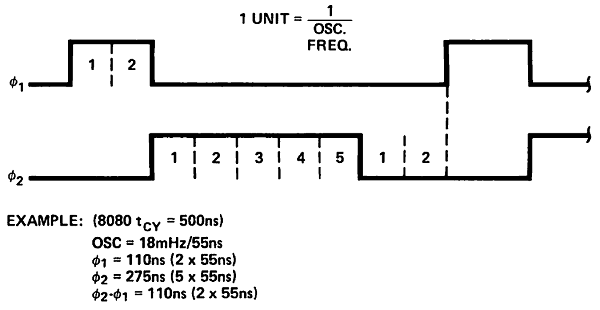Figure 2: 8224 Clock Generator 2-phase clock sequence

In order to build such clock, we can use a mod-9 counter (since there are 9 ticks in a cycle). A mod-9 counter counts from 0 to 8 and then wraps around. Figure 3 shows the schematic of the counter. Note that since the maximum value of 8 requires 4 bits, there are 4 flip flops in the schematic.

Figure 4 below shows the two phase clock circuit using the mod-9 counter. Phase 1 is HIGH when `cnt` is 0 or 1. Otherwise it’s LOW. Phase 2 is HIGH when `cnt` is 2, 3, 4, 5 or 6, otherwise it’s low. The two outputs, `phi1` and `phi2` are used as clock inputs to an i8080 CPU.

Theoretically, everything should work correctly if we use the FF from figure 1. The counter should count from 0 to 8, `phi1` and `phi2` will be calculated based on the counter’s value and the i8080 should function as expected. Unfortunately, this isn’t the case (otherwise I wouldn’t have written this :)). Let’s take a look at the timing output of the circuit from figure 4, where the mod-9 counter has been implemented using the FF from figure 1.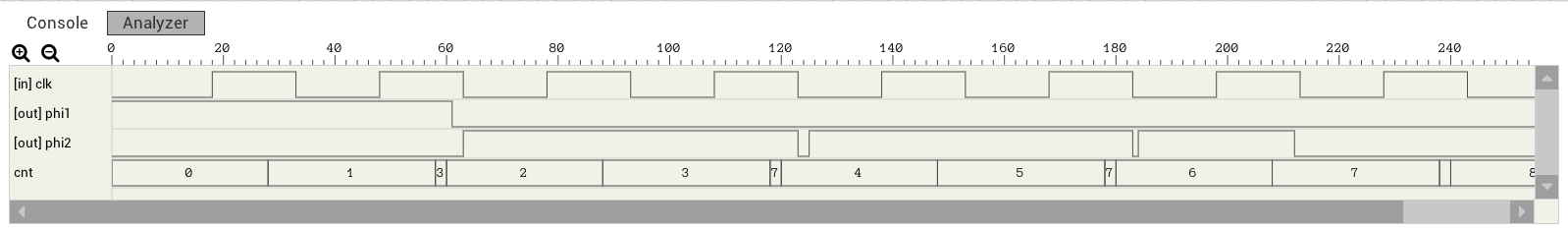Figure 5: Wrong 2-phase clock timing graph

`phi1` looks correct. But `phi2` has 2ns spikes every time the next counter value requires 2 bits to be flipped (e.g. from 1 to 2, bit #0 should go from HIGH to LOW and bit #1 should go from LOW to HIGH). This is because the FF from figure 1 has a different clk-to-Q propagation delay depending on whether Q is going to rise (0 to 1) or fall (1 to 0). Figures 6 and 7 show the critical path for both cases.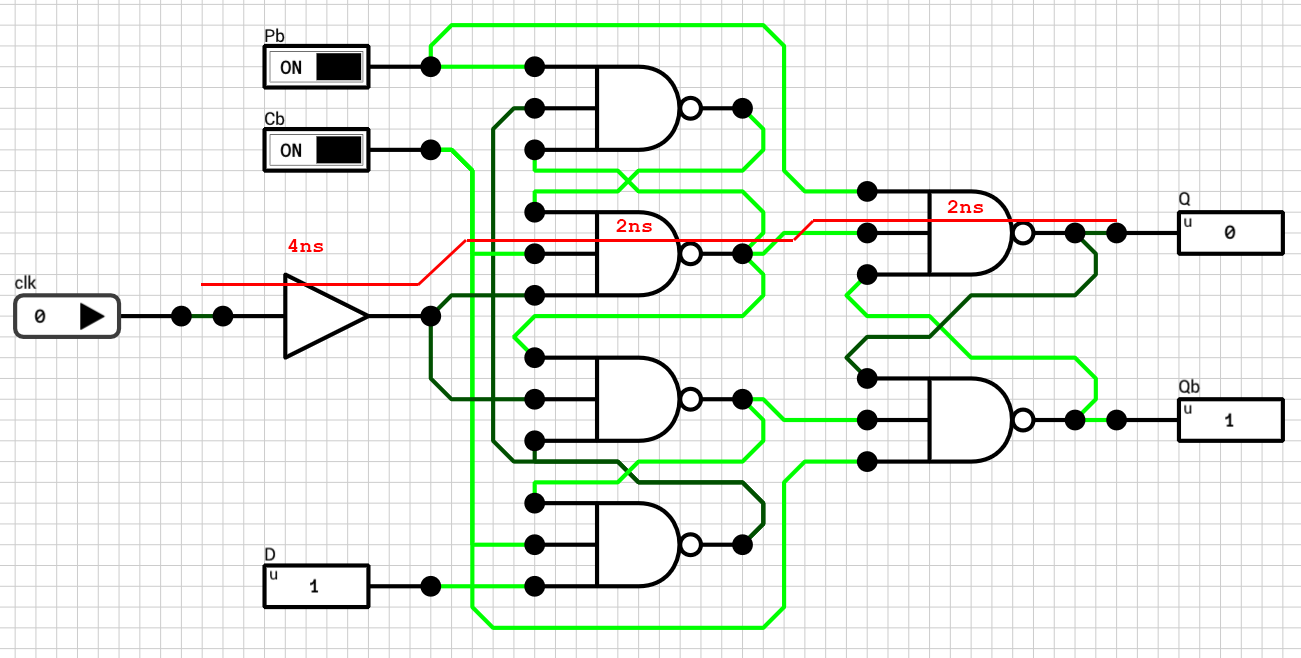Figure 6: D FF critical path for LOW to HIGH transition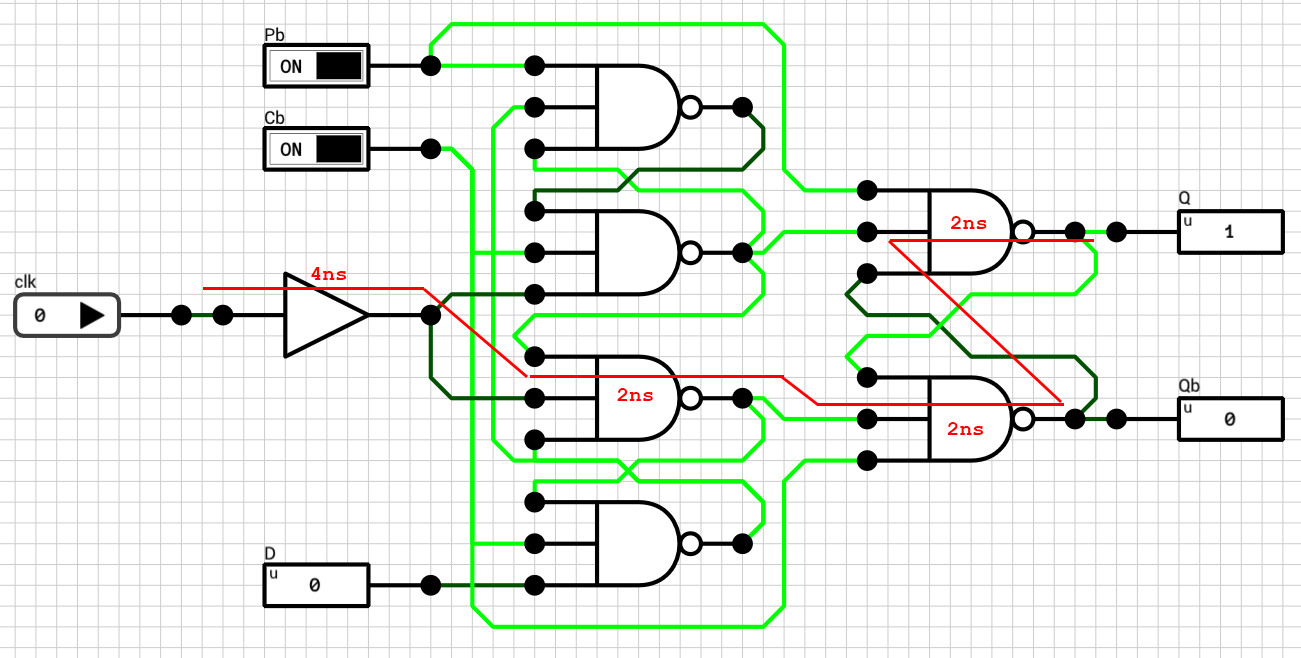Figure 7: D FF critical path for HIGH to LOW transition

When `Q` is going to rise, the rising edge of the `clk` requires 8ns to reach it. When `Q` is going to fall, the `clk` signal requires 10ns to reach it. There is a 2ns difference between the two cases and this is the reason for the spikes in figure 5. The mod-9 counter generates the sequence 0, 1, 3, 2, 3, 7, 4, 5, etc., instead of the expected sequence of 0, 1, 2, 3, 4, 5, etc., because bits go from LOW to HIGH faster than they go from HIGH to LOW.

The fix is easy given the paths from figures 6 and 7. The best we can do is to make the fast path a bit slower. This can be achieved by inserting a 2ns buffer as shown in figure 8.Figure 8: Positive-edge-triggered D flip-flop with equal rising and falling propagation delays

Figure 9 shows the timing graph of the two phase clock using a mod-9 counter implemented with the FF from figure 8.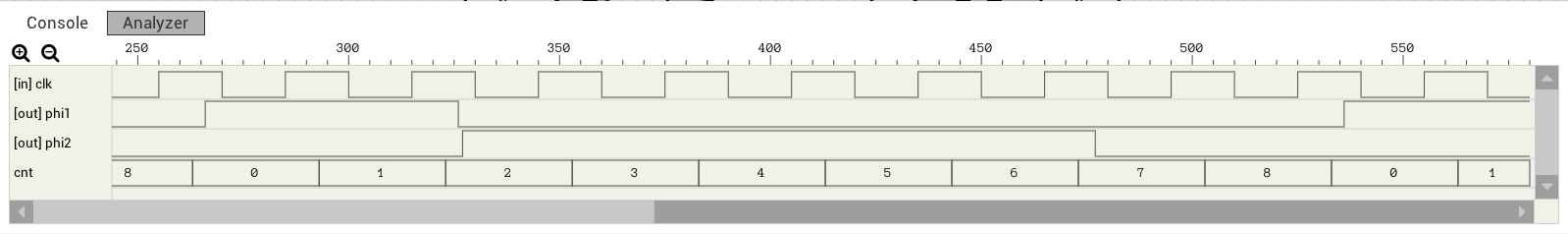Figure 9: Correct 2-phase clock timing graph

Note: The final FF from figure 8 still includes the 4ns buffer after the `clk` which I added in a previous post for the register file. This buffer is used to synchronize `clk` and `D` in case both of them switch at the same time. In other words, it makes the setup time of the FF equal to 0 (tS = 0). In the counter case, this delay shouldn’t be needed. By removing the buffer, the FF’s propagation delay can be reduced to 6ns instead of 10ns. This means that the counter can work with a faster clock.

# Revisiting the Register File

If you happen to be one of the two readers of this blog who have actually checked out the circuits I’ve posted, you might have found out that the register file circuit (RF) I presented in an earlier post doesn’t work quite right. If you tried it out by manually changing the input values (e.g. `rD` and `dD`), everything appears to work correctly. Whenever the clock ticks, the new value is written to the selected register.

The problem I’m going to talk about appears when you try to use this component as part of a larger circuit. If you connect your clock directly to the `clk` input of the RF but the `dD` and `rD` inputs are connected to some other component’s output, you’ll need 2 clock ticks to actually write the new value to the selected register. This is because I didn’t pay attention to propagation delays when designing the various components and the clock signal arrives to the flip flops before the new data signal.

So, let’s fix the circuit by starting from the basic element of the RF, as I did in the original post.

## Positive edge triggered D flip flop

The original DFF I used is shown in figure 1 for reference. Ideally, whenever `clk` goes from LOW to HIGH, the current `D` value is reflected on the `Q` output. Unfortunately, this isn’t true. In order to test it out we need a testbench. Since `D` is an 1-bit signal, there are two transitions to consider: `D` going from LOW to HIGH and vice versa.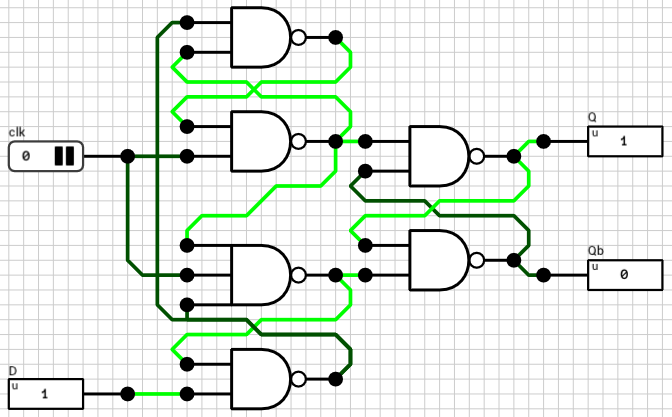Figure 1: The original Positive Edge Triggered D Flip Flop circuit

There are 3 different cases when it comes to the timings of `clk` and `D` inputs.

1. `clk` signal arrives before `D`
2. `clk` signal arrives at the same time as `D`
3. `clk` signal arrives after `D`

Cases 2 and 3 are the ones we are interested in. The 1st case works correctly because if the clock signal arrives before the new data, it means that the controlling circuit wanted to write the old data to the flip flop. In other words, it’s the controlling circuit’s responsibility to synchronize the two signals.

On the other hand, if the rising edge of `clk` arrives after the new `D` value, we must assume that the new data will be written to the flip flop. So, the worst case scenario is that both `clk` and `D` arrive at the exact same time.

In order to find out if the current circuit works as expected, I used a testbench. Testbenches are an easy way to change multiple input values at the same time before triggering a simulation. Script 1 below shows the testbench I used.

``````-- Reset the circuit to a known state
set("clk", 0);
set("D", 0);
simulate();

-- D 0 -> 1
set("D", 1);
tick("clk");
assert(get("Q") == 1, "Failed");
assert(get("Qb") == 0, "Unstable!");
tick("clk");

-- D 1 -> 0
set("D", 0);
tick("clk");
assert(get("Q") == 0, "Failed");
assert(get("Qb") == 1, "Unstable!");
tick("clk");
``````

Script 1: DFF testbench

Initially the circuit is reset to a known state (`clk = 0` and `D = 0`). The first test is for the LOW-to-HIGH `D` transition and the second and final test is for the HIGH-to-LOW transition. Remember that `tick()` toggles the specified clock value and triggers a simulation.

If you execute this testbench in the simulator you’ll find out that the HIGH-to-LOW transition of the `D` signal doesn’t work (the first assert of the second test is triggered and the testbench is terminated). This means that when `D` goes from HIGH to LOW, the time required for the `clk` signal to arrive to the output latch is less than the time required for the new `D` value, which results in the old `D` value being written to it.

In order to fix it, the clock signal should be delayed. The easiest way to delay a signal in the current version of DLS is to use an AND gate. By passing the same signal to all its inputs, you get the same value on its output, at a later (internal) timestep. In DLS, each basic gate has its own propagation delay, which is dependent on the number of inputs (check appendix A of the manual for details). In our case, an AND2 gate has a delay of 1T and an AND3 gate has a delay of 2T.

By trial and error, I found that the required delay for the clock signal is 2T (a single AND3 gate or two AND2 gates in series). The final, corrected, DFF circuit is shown in figure 2.Figure 2: The corrected Positive Edge Triggered D Flip Flop circuit

The testbench works correctly with this circuit. This means that if the controlling circuit sends both signals at the exact same timestep, the flip flop will work correctly. If the clock signal arrives at a later timestep than the `D` signal it will also, by definition, work correctly.

## 1-bit Register

In the same vein, let’s test the original 1-bit register (figure 3). In this case, there’s an extra 1-bit input (`load`) which determines if the new `D` value will be written to the flip flop or not. The testbench used to check this circuit is shown in Script 2.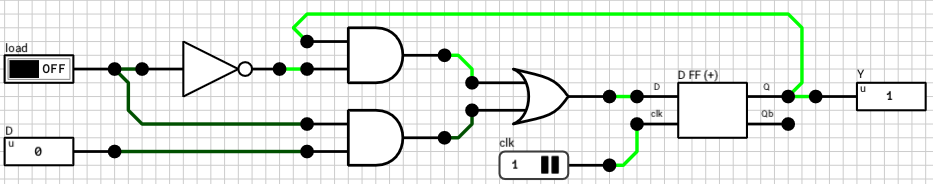Figure 3: The original 1-bit Register
``````-- Reset circuit
set("clk", 0);
set("Din", 0);
simulate();

-- D: 0 -> 1, load: 1
set("Din", 1);
tick("clk");
assert(get("Dout") == 1, "Failed");
tick("clk");

-- D: 1 -> 0, load: 1
set("Din", 0);
tick("clk");
assert(get("Dout") == 0, "Failed");
tick("clk");

-- D: 0 -> 1, load: 1 -> 0
set("Din", 1);
tick("clk");
assert(get("Dout") == 0, "Failed");
tick("clk");

-- D: 1, load: 0 -> 1
tick("clk");
assert(get("Dout") == 1, "Failed");
tick("clk");

-- D: 1 ->, load: 1 -> 0
set("Din", 0);
tick("clk");
assert(get("Dout") == 1, "Failed");
tick("clk");
``````

Script 2: 1-bit Register testbench

The delay of the critical path of the DFF controlling circuit (i.e. the multiplexer in front of the DFF) is 3T (from `load` to OR output). So in order to make `D` and `clk` arrive at the same time to the DFF component, the clock should be delayed by 3T (one AND2 gate and one AND3 gate in series). Figure 4 shows the new 1-bit register circuit which passes all the tests in the testbench.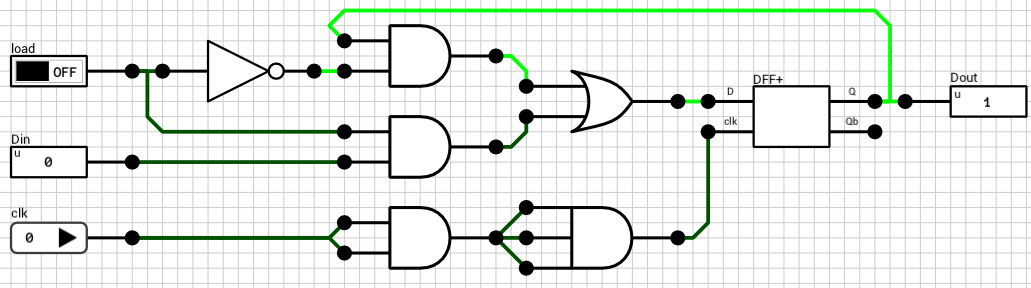Figure 4: The corrected 1-bit Register circuit

## 16-bit Register

Once more, the clock in the 16-bit register circuit should be delayed until the `D` signal is ready to be fed to the 1-bit registers. Only a 16-bit wire splitter exists between `Din` and the 16 1-bit registers and the wire splitter has a delay of 1T (independent of the number of bits). So by delaying the clock signal by 1T, both `Din` and `clk` arrive at the 1-bit registers at the same time. Note that the `load` signal is already split and directly connected to the registers, so it should be valid when `clk` and `Din` arrive.

Script 3 below shows the testbench for the final 16-bit register circuit from figure 5. This time, since the number of possible transistions of the `Din` signal are way too many to exhaust, I used random inputs for the `Din` port.

``````set("clk", 0);
set("Din", 0);
simulate();

local D = randBits(16);
set("Din", D);
tick("clk");
assert(get("Dout") == D, "Failed");
tick("clk");

for i=1, 1000 do
local v = randBits(16);
local load = randBits(2);

set("Din", v);

tick("clk");

local expectedValue = v;
if(load == 0) then
expectedValue = D;
elseif(load == 1) then
local low = bit.band(v, 0x00FF);
local high = bit.band(D, 0xFF00);
expectedValue = bit.bor(low, high);
elseif(load == 2) then
local low = bit.band(D, 0x00FF);
local high = bit.band(v, 0xFF00);
expectedValue = bit.bor(low, high);
else
expectedValue = v;
end

assert(get("Dout") == expectedValue, "Failed");

D = expectedValue;

tick("clk");
end
``````

Script 3: 16-bit Register testbench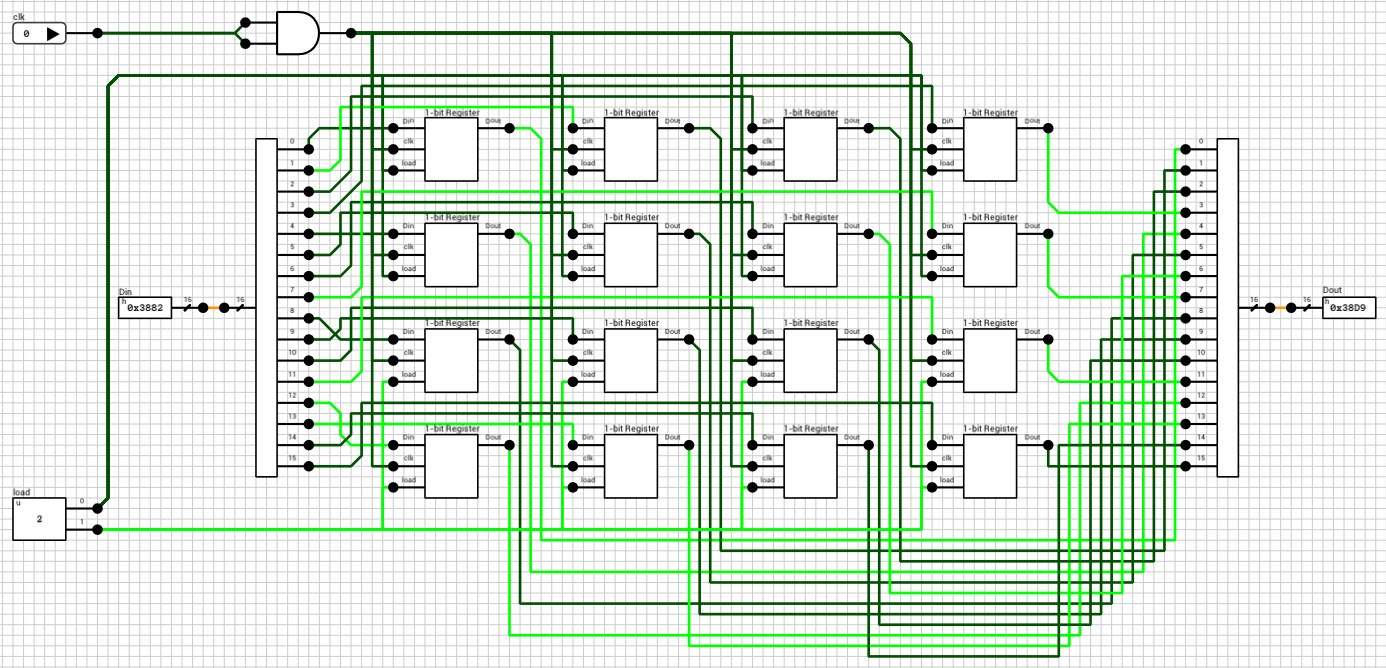Figure 5: The corrected 16-bit Register circuit

## 8x16-bit Register file

Finally it’s time to look the actual register file circuit (figure 6). We are only interested in the write part of the circuit, since reading is performed asynchronously (whenever `rA`, `rB`, `oeA` or `oeB` change, the outputs are immediately updated, without waiting for a `clk` rising edge).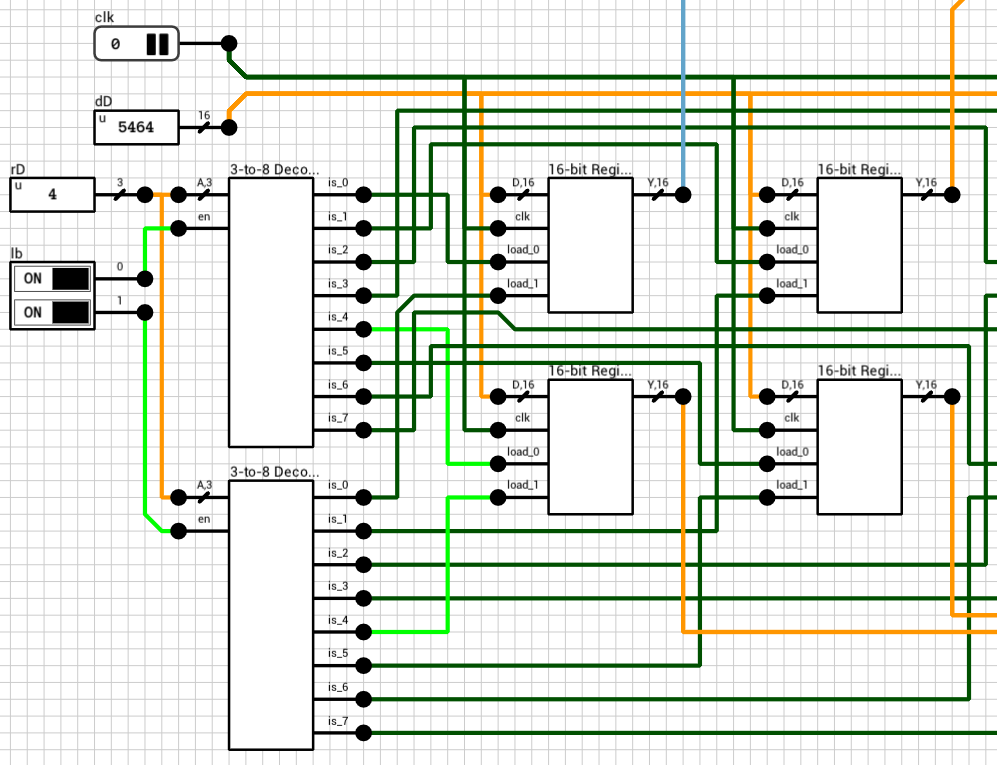Figure 6: The original 8x16-bit Register File circuit (write part)

Both `dD` and `clk` are directly connected to the corresponding inputs of all 8 registers so it’s probably expected that the circuit will work correctly once we replace the old registers with the new components presented above. Script 4 shows a small testbench.

``````-- Reset the circuit. Don't touch dD for extra randomness :)
set("rA", 0);
set("rB", 1);
set("rD", 0);
set("lb", 3); -- Write both bytes to simplify testing
set("clk", 0);
simulate();

-- Test 1: Write a random value to register 0.
local v = randBits(16);
set("dD", v);
tick("clk");
assert(get("dA") == v, "Failed");
tick("clk");

-- Test 2: Write a random value to register 1.
local v2 = randBits(16);
set("dD", v2);
set("rD", 1);
tick("clk");
assert(get("dA") == v, "Failed");
assert(get("dB") == v2, "Failed");
tick("clk");
``````

Script 4: 8x16-bit Register File testbench

As always, the circuit is first reset to a known state. `rA` and `rB` are pointed to registers 0 and 1 respectively, `rD` (the destination register) is set to 0 and `lb` is set to 3, meaning both bytes will be written, to simplify testing.

Test 1 tries to write a new random value to register 0. What’s expected is that when `clk` rises, the new value should be written to the register and `dA` should be updated to reflect it. This works correctly, since the registers have been corrected to handle both signals arriving at the same time.

The 2nd test tries to write another random value to register 1, by switching `rD` to 1 and `dD` to the new value, at the same timestep. It’s expected that when `clk` rises, the new value should be written to the register and `dB` should be updated to reflect it. Unfortunately, this part doesn’t work correctly!

The reason is that there’s a delay on the `load` inputs of each register. By the time `clk` and `dD` arrive at the registers, the old `rD` is used to select the destination, because the 3-to-8 decoder haven’t had a chance to calculate its new output yet.

Looking at the 3-to-8 decoder (figure 7), the critical path delay is 4T, from `A` to `is` (1T for the wire splitter, 1T for the NOT gates and 2T for the AND4 gates). So, delaying the `clk` signal by 4T should do the trick.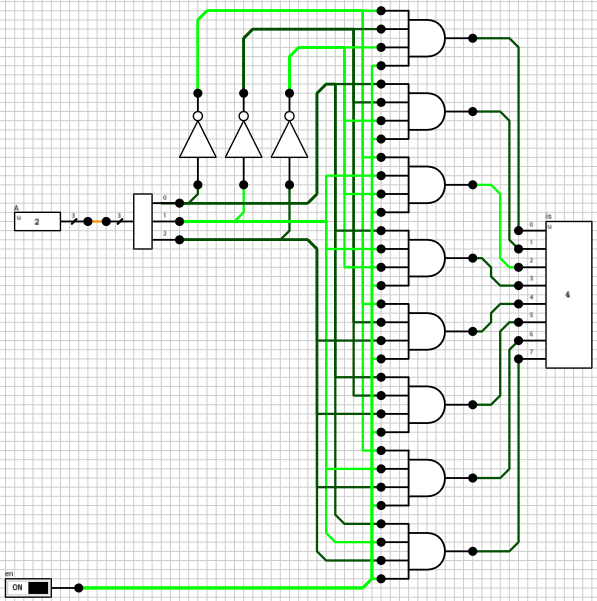Figure 7: Gated 3-to-8 decoder circuit

Figure 8 shows the final register file circuit. The 4T delay has been added to the `clk` signal using two AND3 gates.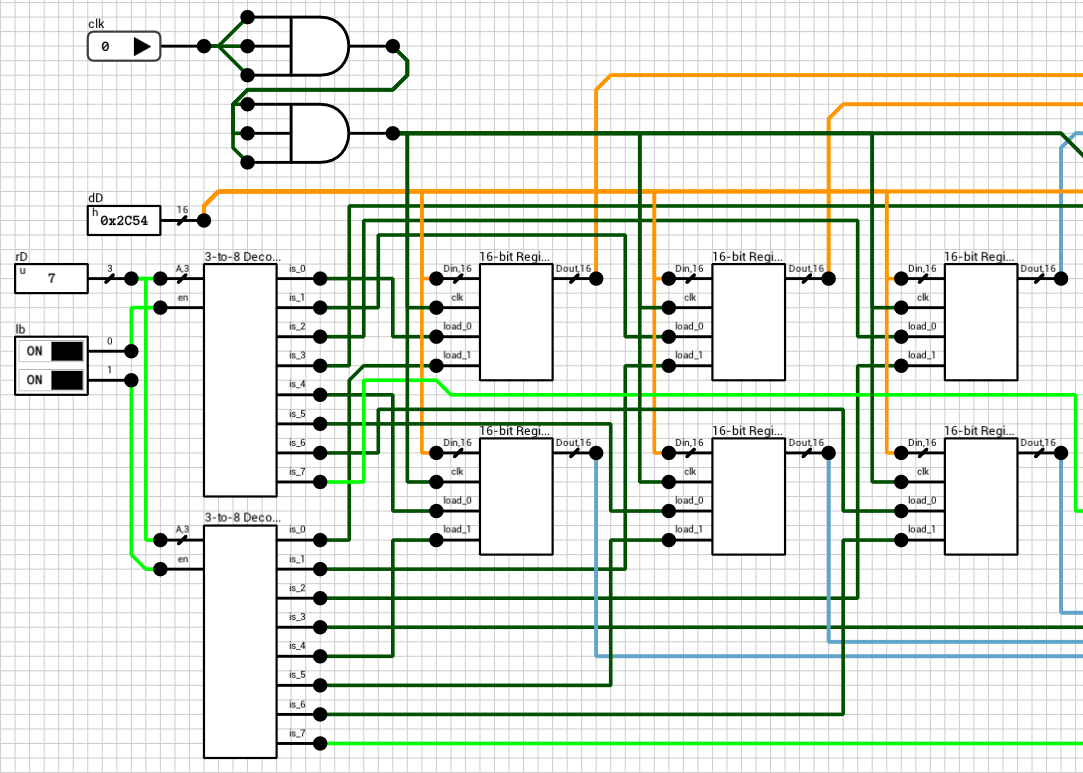Figure 8: The corrected 8x16-bit Register File circuit (write part)

## Conclusion

If there’s something to keep in mind from this post is that whenever there’s a register/flip flop in a circuit, you should make sure that clock’s rising or falling edge arrives to it at the same time or after the data signal. Otherwise, you might need an extra clock cycle to actually store the new value in the register.

Note that the old version worked correctly in all other aspects. It just needed an extra rising edge to actually write the new value to the registers, which sometimes might be annoying when trying to debug it. Having the register file behave in the way we did in this post will make things a bit easier to debug when this component is used in a larger circuit.

Until the next post, comments/suggestions/corrections are welcome.

# 8x16-bit Register File

The goal of today’s post is to build a 8x16-bit register file, i.e. a bunch of memory elements packaged as a single component. Figure 1 shows the final component we are going to build. It can read two registers asynchronously and write one of them on the rising edge of the supplied clock.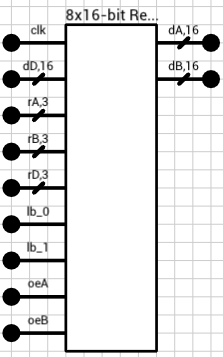Figure 1: 8x16-bit Register File component

The first input is the clock (`clk`). The 16-bit value `dD` will be written to the register pointed by `rD` on the rising edge of the `clk` input. Since the registers will be 2 bytes wide, each byte can be independently written via the `lb_0` and `lb_1` inputs (`lb` = load byte).

Inputs `rA` and `rB` are the two registers we are interested in reading. Their current value will be available on the `dA` and `dB` outputs. Note that these 2 won’t be synchronized to the `clk` input. They can change at any moment and their respective output will be updated (almost) immediately.

Finally, `oeA` and `oeB` inputs (`oe` = output enable) control whether the corresponding output will hold the current value of the selected register, or if it will float. This way, we can use multiple instances of this register file to increase the number of available registers in a circuit, by combining all their outputs on a common bus. More on this at the end of the post.

## Positive edge triggered D flip flop

Let’s start small. The basic building block for this component is the “Positive Edge Triggered D Flip Flop” shown in figure 2. It consists of three cross-coupled active LOW SR latches. Every time the `clk` input goes from LOW to HIGH, `Q` is updated to mirror the input `D`.Figure 2: Positive Edge Triggered D Flip Flop circuit

`Qb` is the complement of `Q`. It won’t be used later, but it’s there for completeness. The same flip flop can be used to build other kinds of circuits where `Qb` might be needed. The latches are connected in a way that the `Q` output is updated only on the transition of the `clk` input from LOW to HIGH (positive edge). In all other cases, no change on the `D` input will affect the `Q` output. For additional details take a look at the wikipedia article on flip flops (paragraph “Classical positive-edge-triggered D flip-flop”).

## 1-bit Register

The flip flop from figure 2 can hold 1 bit of data. The `clk` input doesn’t need to be an actual clock. Any kind of 1-bit input can be used as a clock and it will be updated on its rising edge. In cases where the `clk` input is actually a free-running clock, we might have a problem if we don’t want to update its contents on the next rising edge. E.g. the wire connected to the `D` input changes value but we don’t want to store it in the flip flop on the next clock tick.

Figure 3 shows how this can be accomplished. By using a 2-input mutliplexer in front of the `D` input, with the first MUX choice being the old value and the second choice being the new value, we can select whether we want to update the flip flop or not, via the new `load` input.

In order to distinguish this circuit from the flip flop shown above, I’ll call it an 1-bit Register.

Side note: I’ve created the mutliplexer using 2 AND and 1 OR gates instead of 2 tristate buffers and a 2x1-bit bus, as shown in the ALU post. This is because the tristate MUX has a small glitch which affects the rest of the circuit (at least in DLS). When changing the `sel` input of the tristate MUX, there’s a simulation timestep where both bus inputs are active at the same time. In such cases the buses in DLS are configured to output an `Error` value. If the output of the bus is connected to the `D` input of the flip flop, we might end up in an invalid state, from which it’s impossible to get out of. In the ALU circuit this wasn’t a problem since all components were combinational and they correctly handled `Error` inputs.

## 16-bit Register

Expanding to 16 bits is easy. We just use 16 instances of the 1-bit Register component, wire everything together and we are done. Figure 4 shows the 16-bit Register circuit.

As I mentioned at the beginning of the post, since the register is 16 bits wide (2 bytes), we might want to control (write) each individual byte separately. This is the reason the `load` input is 2 bits wide. Each bit controls one of the bytes. If it’s not obvious from the figure, `load_0` is connected to the `load` inputs of the first 8 1-bit registers and `load_1` is connected to the `load` input of the other 8 1-bit registers.

Side note: The first time a register is initialized, both bytes should be written. The initial state of the D flip flop produces an `Undefined` output (because the `clk` hasn’t ticked yet). Since we don’t mask/split the register output to separate the individual bytes, having only 8 of the 16 bits initialized and the rest in an `Undefined` state will produce an `Undefined` value on the `Y` output. This is because, if at least 1 of the inputs on a wire merger is equal to `Undefined` or `Error`, the rest of the bits are ignored and the special state is propagated to the output.

## The Register File

With the 16-bit Register component ready, we can now build a small register file. To keep things as simple as possible, we’ll use only 8 registers and (as mentioned in the intro) we’ll add a way to mask the outputs in order to be able to cascade multiple instances of this circuit to build larger files. Figure 5 shows the complete circuit. It might be a bit difficult to read, so I’ll break it up into parts, with zoomed in screenshots.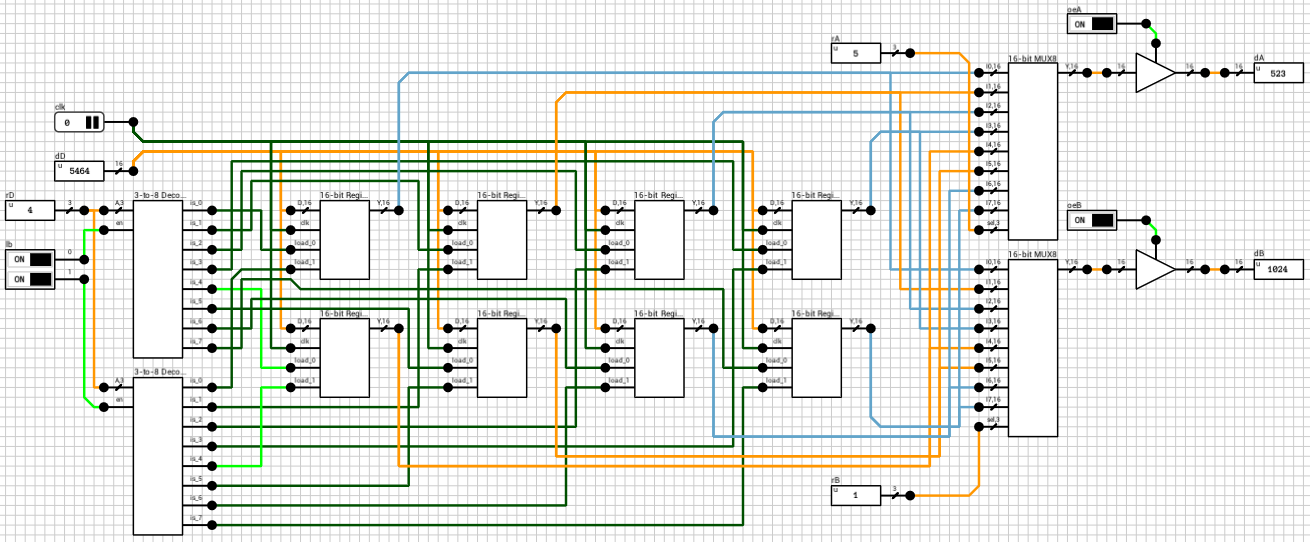Figure 5: 8x16-bit Register File circuit

Figure 6 shows the write part of the circuit. `clk` is the clock and it’s routed to all the `clk` inputs of the 8 registers. `dD` is the 16-bit value we want to write to the `rD` register and it is again connected to the `D` inputs of all the registers. The 3-bit `rD` input is decoded using gated 3-to-8 decoders, one for each byte, based on the `lb` input. A gated decoder (figure 7) works the same way as the decoder we saw in the ALU post, with the only difference being that when its `en` input is LOW, all outputs are LOW.Figure 6: The *write* part of the circuitFigure 7: 3-to-8 Gated Decoder

Figure 8 shows the read part of the circuit. All register outputs are routed to two 16-bit 8-input mutliplexers (figure 9). `rA` and `rB` are used as the `sel` input to the two MUXes. MUX outputs are connected to 16-bit tristate buffers, with the control pins connected to the `oeA` and `oeB` inputs.

Note that in this case, since the MUX is after the flip flops, we can use tristate buffers. As long as the output of the register file isn’t connected to another register, there shouldn’t be a problem. If there is, we can always come back and replace the MUX with an AND/OR version.

## Larger register files

The component presented above (figure 5) can be used to build both wider and deeper register files. Unfortunately, DLS doesn’t currently support bit widths larger than 16 bits per wire/pin, so building (e.g.) a 32-bit register file will end up in a mess of wires :) It’s possible, but you’ll need double the IO pins and wires.

Instead of building a wider file we’ll build a larger/deeper one, with 16 registers, using two instances of the component. In this case `rA`, `rB` and `rD` should be expanded to 4 bits, with their MSB used to select the correct file, by turning on or off the corresponding `load` and `oe` inputs. The `dA` and `dB` outputs of the two instances are connected on one bus each and then routed to the final outputs. Figure 10 shows the final 16x16-bit register file circuit.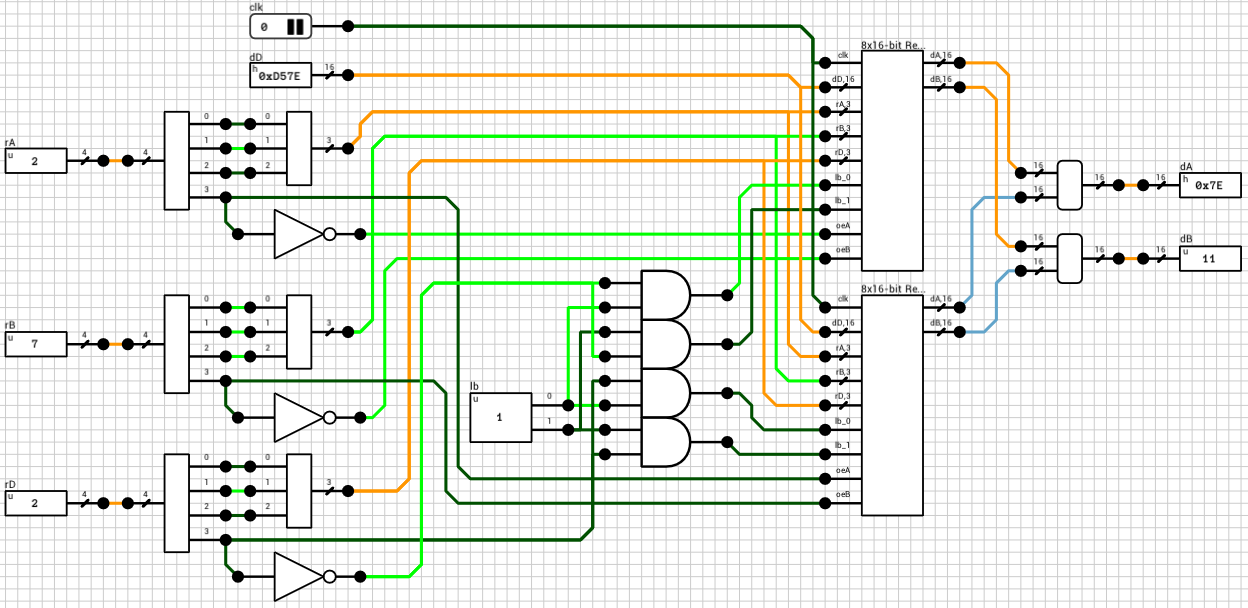Figure 10: 16x16-bit Register File circuit

Note that in this circuit there’s no output enable inputs since I assumed this component won’t be used to build even larger components. If this is the case, both `oe` inputs should be exposed to correctly handle cascading.

That’s all for now. Thanks for reading. As always, comments and corrections are welcome.